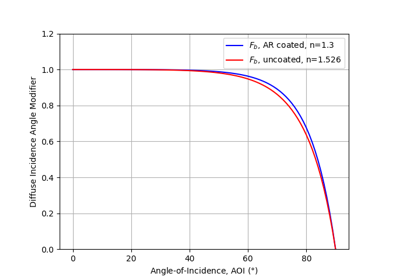# pvlib.iam.physical#

pvlib.iam.physical(aoi, n=1.526, K=4.0, L=0.002, *, n_ar=None)[source]#

Determine the incidence angle modifier using refractive index n, extinction coefficient K, glazing thickness L and refractive index n_ar of an optional anti-reflective coating.

iam.physical calculates the incidence angle modifier as described in 1, Section 3, with additional support of an anti-reflective coating. The calculation is based on a physical model of reflections, absorption, and transmission through a transparent cover.

Parameters
• aoi (numeric) – The angle of incidence between the module normal vector and the sun-beam vector in degrees. Angles of nan will result in nan.

• n (numeric, default 1.526) – The effective index of refraction (unitless). Reference 1 indicates that a value of 1.526 is acceptable for glass.

• K (numeric, default 4.0) – The glazing extinction coefficient in units of 1/meters. Reference  indicates that a value of 4 is reasonable for “water white” glass.

• L (numeric, default 0.002) – The glazing thickness in units of meters. Reference 1 indicates that 0.002 meters (2 mm) is reasonable for most glass-covered PV panels.

• n_ar (numeric, optional) – The effective index of refraction of the anti-reflective (AR) coating (unitless). If n_ar is None (default), no AR coating is applied. A typical value for the effective index of an AR coating is 1.29.

Returns

iam (numeric) – The incident angle modifier

Notes

The pvlib python authors believe that Eqn. 14 in 1 is incorrect, which presents $$\theta_{r} = \arcsin(n \sin(AOI))$$. Here, $$\theta_{r} = \arcsin(1/n \times \sin(AOI))$$

References

1(1,2,3,4)

W. De Soto et al., “Improvement and validation of a model for photovoltaic array performance”, Solar Energy, vol 80, pp. 78-88, 2006.

2

Duffie, John A. & Beckman, William A.. (2006). Solar Engineering of Thermal Processes, third edition. [Books24x7 version] Available from http://common.books24x7.com/toc.aspx?bookid=17160.

## Examples using pvlib.iam.physical#Diffuse IAM Calculation

Diffuse IAM Calculation